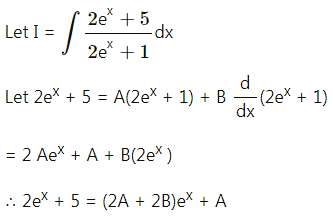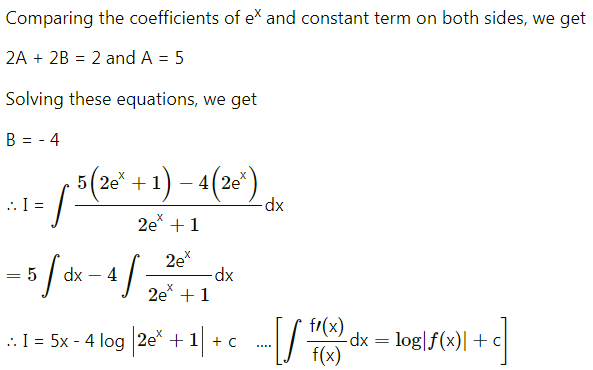Balbharati Maharashtra State Board Std 12 Commerce Statistics Part 1 Digest Pdf Chapter 5 Integration Ex 5.3 Questions and Answers.

## Maharashtra State Board 12th Commerce Maths Solutions Chapter 5 Integration Ex 5.3

Evaluate the following:

Question 1.
$$\int \frac{3 e^{2 t}+5}{4 e^{2 t}-5} d t$$
Solution:
Let I = $$\int \frac{3 e^{2 t}+5}{4 e^{2 t}-5} d t$$
Put, Numerator = A(Denominator) + B[$$\frac{d}{d x}$$(Denominator)]
∴ 3e2t + 5 = A(4e2t – 5) + B[$$\frac{d}{d t}$$(4e2t – 5)]
∴ 3e2t + 5 = A(4e2t – 5) + B[4e2t × 2 – 0]
∴ 3e2t + 5 = (4A + 8B) e2t – 5A
Equating the coefficient of e2t and constant on both sides, we get
4A + 8B = 3
and -5A = 5
∴ A = -1
∴ from (1), 4(-1) + 8B = 3
∴ 8B = 7
∴ B = $$\frac{7}{8}$$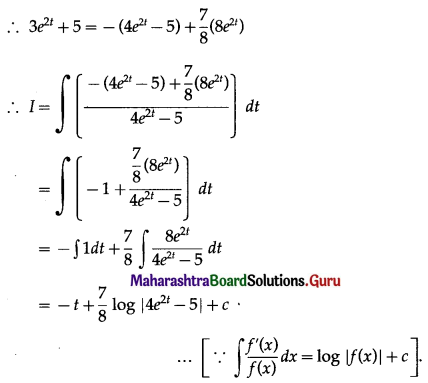Question 2.
$$\int \frac{20-12 e^{x}}{3 e^{x}-4} d x$$
Solution:
Let I = $$\int \frac{20-12 e^{x}}{3 e^{x}-4} d x$$
Put, Numerator = A (Denominator) + B[$$\frac{d}{d x}$$(Denominator)]
∴ 20 – 12ex = A(3ex – 4) + B[$$\frac{d}{d x}$$(3ex – 4)]
∴ 20 – 12ex = A(3ex – 4) + B(3ex – 0)
∴ 20 – 12ex = (3A + 3B)ex – 4A
Equating the coefficient of ex and constant on both sides, we get
3A + 3B = -12 ……(1)
and -4A = 20
∴ A = -5
from (1), 3(-5) + 3B = -12
∴ 3B = 3
∴ B = 1
∴ 20 – 12ex = -5(3ex – 4) + (3ex)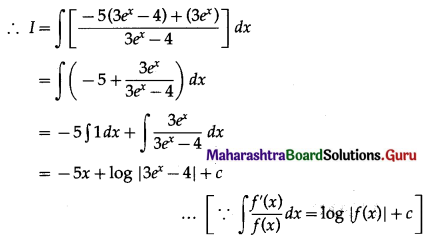Question 3.
$$\int \frac{3 e^{x}+4}{2 e^{x}-8} d x$$
Solution:
Let I = $$\int \frac{3 e^{x}+4}{2 e^{x}-8} d x$$
Put, Numerator = A (Denominator) + B[$$\frac{d}{d x}$$(Denominator)]
∴ 3ex + 4 = A(2ex – 8) + B[$$\frac{d}{d x}$$(2ex – 8)]
∴ 3ex + 4 = A(2ex – 8) + B(2ex – 0)
∴ 3ex + 4 = (2A + 2B)ex – 8A
Equating the coefficient of ex and constant on both sides, we get
2A + 2B = 3 ……..(1)
and -8A = 4
∴ A = $$-\frac{1}{2}$$
∴ from (1), 2($$-\frac{1}{2}$$) + 2B = 3
∴ 2B = 4
∴ B = 2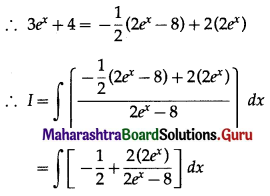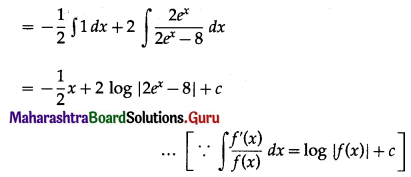Question 4.
$$\int \frac{2 e^{x}+5}{2 e^{x}+1} d x$$
Solution: